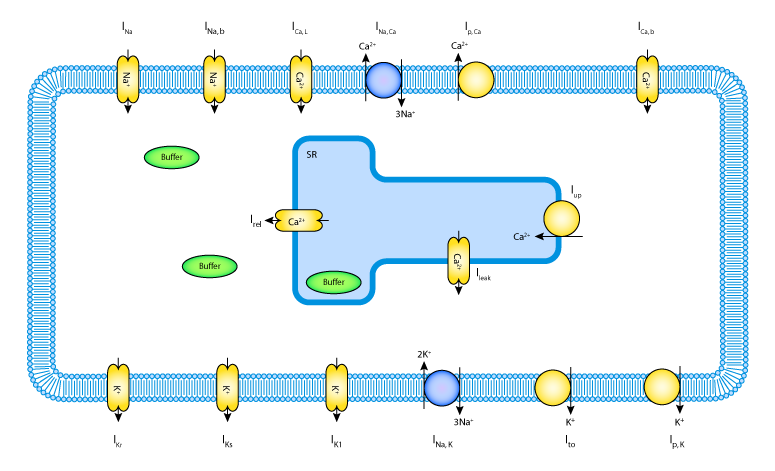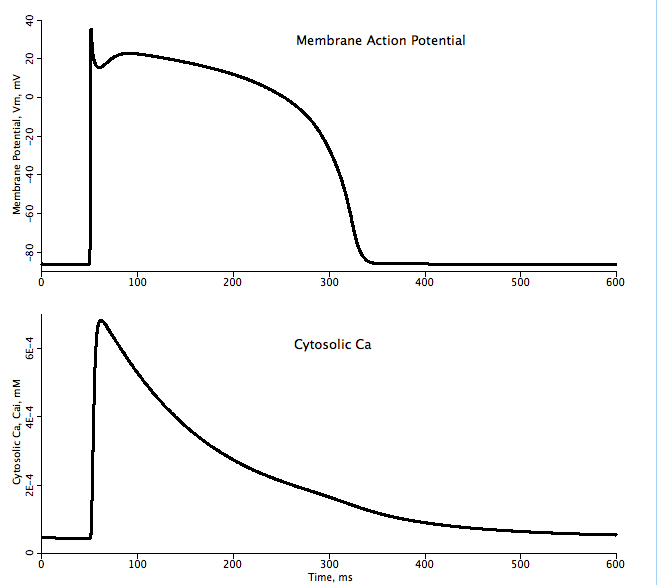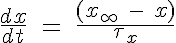# ten_Tusscher_Noble_Panfilov2004

Model number
0201

This model simulates the action potential for a human ventricular myocyte accounting for Na, K and Ca transport and dynamics.

## Description

```   This model simulates the electrophysiology of a single cardiomyocyte taking into
consideration selected Na, K and Ca transport along with Ca shuttling between the
sarcoplasmic reticulum (SR) and cytosol, and buffering of Ca in both cytosol and
SR.  The model is based on the study by ten Tusscher et al. (Amer J Physiol 286:
JSim model from:

www.physiome.org/jsim/models/cellml/#tentusscher_noble_noble_panfilov_2004

and modifying it to run in with unit checking enabled.  A comparison to the
same model running in the CellML simulation suite PCEnv which was downloaded from:

www.cellml.org/models/tentusscher_noble_noble_panfilov_2004

enabled the following changes to be made:

1) The membrane capacitance is not used in the equation for dV/dt since
the currents are already given in units of pA/pF which would indicate
capacitance has already been factored in.  Therefore the expression used in
this simulation is:

dV/dt = -1 * ( i_ion + i_stim )

dV/dt = -1 * ( i_ion + i_stim ) / Cm

which is given in the original paper.

2) Membrane reversal potentials are incorrectly presecribed as log(Co/Ci)
(base 10) instead of ln(Co/Ci) in the CellML version 1 that is used
to generate the JSim project file.

3) A different expression is used for the rate of change of gating parameters
used for fast Na m, h and j gate, L-type Ca d gate, slow delayed rectifier
K Xs gate, rapid delayed rectifier K Xr1 and Xr2 gate.  In CellML version
1 they were specified as:

dx/dt = alpha_x * (1 - x) + beta_x * x

while in the PCEnv version

dx/dt = (x_infinity - x ) / tau_x

is used where x is the gating variable

4) Error in the Ca dependent inactivation gate for L-type Ca channel
calculation involving alpha_fCa.  In version 1 used for JSim:

alpha_fCa = 1 / (1 + exp((Cai / (3.25E-4 mM))^8))

whereas in the PCEnv version:

alpha_fCa = 1 / (1 + ((Cai / (3.25E-4 mM))^8))

is used.

5) Incorporation of different Ca dynamics expressions that existed in the
PCEnv version but were not documented in the original paper or used in
the JSim version.  In the paper buffering was calculated but did not
factor in the calcium dynamics.  Also in order to convert the Ca currents
into Ca fluxes the membrane capacitance must be included but was not. The
equations out of the paper and used in CellML version 1 are:

Cai_bufc = Cai * Bufc / (Cai + Kbufc)
Casr_bufsr = CaSR * Bufsr / (CaSR + Kbufsr)
Cai:time = ((i_CaL + i_bCa + i_pCa - (2*i_NaCa)) / (2*Vc*F))
+ i_leak - i_up + i_rel;
CaSR:time = (Vc/Vsr) * (i_up - i_rel - i_leak)

where the following equations are used in PCEnv:

Cai_bufc = 1 / (1 + (Bufc * Kbufc) / ((Cai + Kbufc)^2))
Casr_bufsr = 1 / (1 + (Bufsr * Kbufsr)/ ((CaSR + Kbufsr)^2))
Cai:time = Cai_bufc * (i_leak - i_up + i_rel
- Cm  * ((i_CaL + i_bCa + i_pCa - (2*i_NaCa)) / (2*Vc*F)))
CaSR:time = Casr_bufsr * (Vc/Vsr) * (i_up - i_rel - i_leak)

6) Also Na and K dynamics were altered to take into consideration the
membrane capacitance.  The CellML version 1 and original paper
equations are:

Nai:time = -1 * (i_Na + i_bNa + (3*i_NaK)
+ (3*i_NaCa)) / (Vc*F)
Ki:time = -1 * (i_K1 + i_to + i_Kr + i_Ks + i_pK
+ i_stim + i_pK - (2*i_NaK) - i_ax) / (Vc*F)

where the PCEnv simulation uses

Nai:time = -Cm * (i_Na + i_bNa + (3*i_NaK)
+ (3*i_NaCa)) / (Vc*F)
Ki:time = -Cm * (i_K1 + i_to + i_Kr + i_Ks + i_pK
+ i_stim - (2*i_NaK)) / (Vc*F)```## EquationsEquations for this model are presented in the AJP article with the following additions and exceptions:

1. Currents are represented in pA/pF therefore membrane capacitance has already been taken into account in the expression for dV/dt
2. Calcium dynamics equations have been changed to include dependence on buffering.
3. Ca, Na and K dynamics now account for membrane capacitance.
4. The rate of change of all gating variables is:unless indicated otherwise in paper.

The parameters and equations used in this model are for the epicardial myocyte variant to match the graphs given in Figure 7.

For more details please look at notes in JSim model source code.

The equations for this model may be viewed by running the JSim model applet and clicking on the Source tab at the bottom left of JSim's Run Time graphical user interface. The equations are written in JSim's Mathematical Modeling Language (MML). See the Introduction to MML and the MML Reference Manual. Additional documentation for MML can be found by using the search option at the Physiome home page.

References
``` ten Tusscher KHWJ, Noble D, Noble PJ and Panfilov AV;
A model for human ventricular tissue, Amer J Physiol 286:H1573-H1589, 2004

Luo C and Rudy Y; A dynamic model of the cardiac ventricular action potential.
I. simulations of ionic currents and concentration changes, Circ Res 74:1071-1096, 1994.```
Key terms
Human
ventricular
myocyte
electrophysiology
Publication
Cell Physiology
Action potential
PMID14656705
Acknowledgements

Please cite https://www.imagwiki.nibib.nih.gov/physiome in any publication for which this software is used and send one reprint to the address given below:
The National Simulation Resource, Director J. B. Bassingthwaighte, Department of Bioengineering, University of Washington, Seattle WA 98195-5061.

Model development and archiving support at https://www.imagwiki.nibib.nih.gov/physiome provided by the following grants: NIH U01HL122199 Analyzing the Cardiac Power Grid, 09/15/2015 - 05/31/2020, NIH/NIBIB BE08407 Software Integration, JSim and SBW 6/1/09-5/31/13; NIH/NHLBI T15 HL88516-01 Modeling for Heart, Lung and Blood: From Cell to Organ, 4/1/07-3/31/11; NSF BES-0506477 Adaptive Multi-Scale Model Simulation, 8/15/05-7/31/08; NIH/NHLBI R01 HL073598 Core 3: 3D Imaging and Computer Modeling of the Respiratory Tract, 9/1/04-8/31/09; as well as prior support from NIH/NCRR P41 RR01243 Simulation Resource in Circulatory Mass Transport and Exchange, 12/1/1980-11/30/01 and NIH/NIBIB R01 EB001973 JSim: A Simulation Analysis Platform, 3/1/02-2/28/07.